Operations

Sum of the numbers 1.01 and 3.35 multiply by the difference of numbers 6.69 and 1.39.

Result

x =  23.11

Solution:Leave us a comment of this math problem and its solution (i.e. if it is still somewhat unclear...):Be the first to comment!Next similar math problems:

1. Product of the sum and differenceCalculate the product of the sum and difference of numbers -7 and -2.
2. With bracket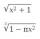Calculate (evaluate) simple mathematical expression with a negative numbers and a bracket: 13+15*5-2*(-6)
3. ProductResult of the product of the numbers 1, 2, 3, 1, 2, 0 is:
4. How manzBy how many is the product of the numbers 328 and 7 greater than its sum?
5. Roman numerals 2+Add up the number writtens in Roman numerals. Write the results as a roman numbers.
6. What is missing (1000)What number is to add to get 1000?
7. Icecreams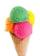Karthik bought 30 icecreams for his birthday .2/5 of them are chocolate icecreams. Find the number of chocolate icecreams he bought?
8. Roman numerals +Add up the number writtens in Roman numerals. Write the results as a decimal number.
9. Decimal expansion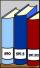Calculate: 2 . 1 + 0 . 10 + 7 . 10000 + 4 . 1000 + 6 . 100 + 0 . 100000 =
10. Expression 1What is 7+8-(5×2)+5-4+(6×(5-3)+6)-(8+10)-7+6?
11. Boys and girls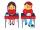In the class are 20 boys and 5-times less girls. How many girls are in the classroom? How many all children are in the class?
12. School trip6.A class went on a trip. Train ticket cost 6 euros, cable car ticket 6 euro. How many euros must pay classroom teacher for 23 students?
13. Stamps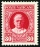Tibor has four times more stamps than Miro and seven times more stamps than Stano. How many stamps have all three if Tibor has 504 stamps?
14. Bus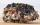On the 4-th stop take on 56 and take off 38 passengers. How many were added (write as positive number) or shrunk (write as negative number) the count of passengers?
15. Car parking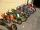The car park has 45 motorcycles and 25 cars. 14 cars went away and 2 arrived, then departs 12 motorcycles and 2 arrive. How many motorbikes and cars there are now?
16. Book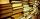Jitka read on holidays book that has 180 pages. In the first week read 45 pages. In the second week she read 15 pages more than the first week. How many pages left to read it yet?
17. Baking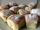There are 28 bunches, and son ate 1/2, dad ate four bunches. How many of them remain on the baking dishes?